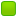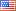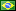###Author Topic: can somebody help me figure out fibonacci recurse?  (Read 10159 times)

####sphinx

• Jr. Member
•• Posts: 2##### can somebody help me figure out fibonacci recurse?
« on: March 16, 2021, 07:12:32 AM »
below is my implementation, when I send 10 to the param, it returns 8.
I can't figure out what's wrong.

`asm
global fib

section .text

fib:
cmp rdi, 1              ; if less equal than 1
jle done                ; return

dec rdi                 ; n-1
push rdi                ; push n-1
call fib                ; calculate f(n-1)
mov rcx, rax            ; get f(n-1) results and save to rcx
pop rdi                 ; restore n-1

dec rdi                 ; n-2
push rdi                ; push n-2
call fib                ; calculate f(n-2)
add rax, rcx            ; calulate f(n-1) + f(n-2)
pop rdi                 ; restore n-2

done:
mov rax, rdi
ret
`

####Frank Kotler

• NASM Developer
• Hero Member
•• Posts: 2565
• Country:##### Re: can somebody help me figure out fibonacci recurse?
« Reply #1 on: March 16, 2021, 11:10:00 PM »
Hi sphinx,

Welcome to the Forum.

The "call" instruction pushes the return address onto the stack. So "pop rdi" gets the return address! Old rdi is at "rsp + 8". So "mov rdi , [rsp + 8] " is probably what you want.

Best,
Frank

####sphinx

• Jr. Member
•• Posts: 2##### Re: can somebody help me figure out fibonacci recurse?
« Reply #2 on: March 17, 2021, 02:01:16 AM »
Thanks for you reply. You said [ "pop rdi" will gets the return address!] , but I found [pop rdi] actually works well.
I am new to NASM or assembly, please make sure you didn't miss something.
after some googling, I figured out what's wrong.
first, I did not save rcx, so its changed during recurse.
second, I forget ret after add f(n-1) and f(n-2).

now it works.

`asm
global fib

section .text

fib:
cmp rdi, 1              ; if less than 1
jle done                ; return

; calculate f(n-1)
dec rdi                 ; n-1
push rdi                ; push n-1
call fib                ; calculate f(n-1)
mov rcx, rax            ; get f(n-1) results and save to rcx
pop rdi                 ; restore n-1

; calculate f(n-2)
push rcx                ; save rcx
dec rdi                 ; n-2
push rdi                ; push n-2
call fib                ; calculate f(n-2)
pop rdi                 ; restore n-2
pop rcx                 ; restore rcx
add rax, rcx            ; calulate f(n-1) + f(n-2)
ret

done:
mov rax, rdi
ret
`

####Frank Kotler

• NASM Developer
• Hero Member
•• Posts: 2565
• Country:##### Re: can somebody help me figure out fibonacci recurse?
« Reply #3 on: March 17, 2021, 02:27:36 AM »
Okay. My memory's all shot. Sorry.

Best,
Frank

####fredericopissarra

• Full Member
•• Posts: 202
• Country:##### Re: can somebody help me figure out fibonacci recurse?
« Reply #4 on: March 17, 2021, 12:07:55 PM »
A little better approach:
Code: [Select]
`; fib.asm  bits  64  default rel  section .text  global fib; unsigned int fib( unsigned int n );;; Input: EDI = n (n >= 1); Output: EAX (fibonacci number).  align 4fib:  ; Preserve RBP and RBX (SysV ABI).  push  rbp  push  rbx  mov ebp, 1  cmp edi, 3  jb  .exit  mov ebx, edi  mov ebp, 1  align 4.loop:  lea   edi, [rbx - 1]  call  fib  add   ebp, eax      ; Accumulate.  sub   ebx, 2  ja    .loop.exit:  mov eax, ebp  ; Restore RBP and RBX (SysV ABI).  pop rbx  pop rbp  ret`Test code:
Code: [Select]
`/* test.c */#include <stdio.h>extern unsigned int fib( unsigned int );int main( void ){  unsigned int i;  for ( i = 1; i <= 10; i++ )    printf( "%u ", fib(i) );  putchar( '\n' );}`Compiling, linking and testing:
Code: [Select]
`\$ cc -c -o test.o test.c\$ nasm -felf64 -o fib.o fib.asm\$ cc -o test test.o fib.o\$ ./test1 1 2 3 5 8 13 21 34 55`
« Last Edit: March 17, 2021, 12:12:12 PM by fredericopissarra »

SMF spam blocked by CleanTalk